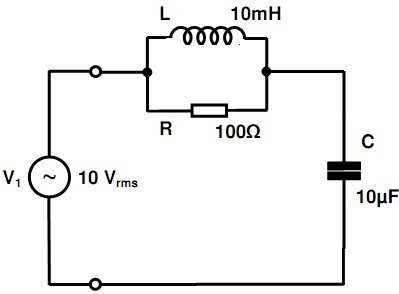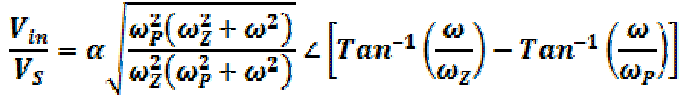Find out the impedance, phase and magnitude

Find out the impedance of the network demonstrated below as considered by the voltage source, V1, while operating at a frequency of 1 kHz. Express it in the form of phase and magnitude. Moreover, find the current drawn by the source by the network in similar form and draw a phasor diagram demonstrating the relationship between given current as well as the source voltage.#### Related Questions in Electrical Engineering

• ##### Q :What is warping effect Explain what is

Explain what is meant by the term warping effect?

• ##### Q :Prove phase shift is similar at pole

The steady state transfer function of the certain electrode amplifier interface model demonstrated below is specified as:##### Q :Define the term CAN bus its used Define

Define the term CAN bus its used?

• ##### Q :What is propagation delay Explain what

Explain what do you mean by the propagation delay? Describe it in detail?

• ##### Q :Meaning of 3 dB cutoff frequency

Illustrate the meaning of 3 dB cutoff frequency? Explain why it is3 dB, not 1 dB?

• ##### Q :Complexity of deriving test of circuit

Explain the factors which measures the complexity of deriving the test of circuit?

• ##### Q :Addressing modes supported by C5X

Explain the addressing modes which are supported by the C5X processors?

• ##### Q :What is SCON Define the term SCON?

Define the term SCON?

• ##### Q :Illustrates software tools of embedded

Illustrates the software tools of an embedded system in designing?

• ##### Q :Demodulation and Stability Define: a)

Define:

a) Demodulation.

b) Stability.